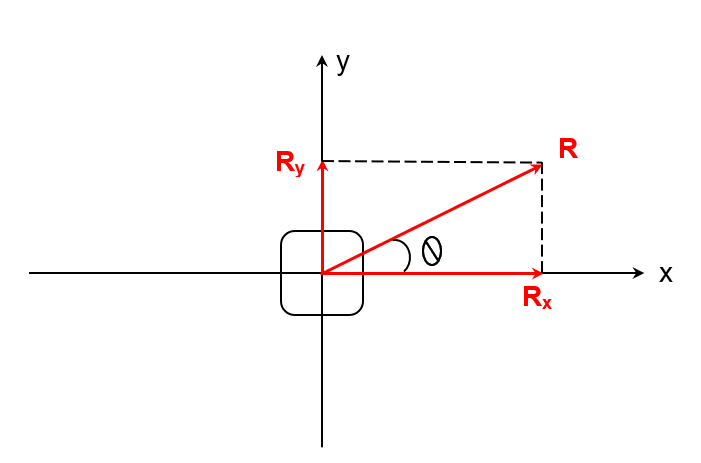# Resultant Force Calculator

Enter the magnitudes and directions of x and y forces in the magnitude of the resultant forces calculator and hit the calculate button.

N
°
N
°## Resultant force Calculator

The resultant force calculator finds the magnitude and the direction of the resultant force. You can find the resultant for up to 2 force vectors.

If you have three vectors e.g a, b, and c, first enter the a and b vectors and then add the resultant vector of a and b and the c vector.

## What is the resultant force?

The resultant force is the net force acting on an object that is under multiple forces. Since force is a vector quantity, the resultant force has both magnitude and direction.

The resultant force can be displayed in the vector form as follows:## Resultant force formula:

There are different formulas for both magnitude and direction.

For magnitude:For magnitude, it is important to know the angle between the vectors.

And for direction/angle:Typically for finding the magnitude, the angle is calculated first.

## How to find the resultant force?

Example:

A car is being pushed by 10 newtons in the forward direction (0o) and by 7 newtons from a 90 degrees angle.

Solution:

Step 1: Identify the values.

Magnitude of F1 = 10N

Magnitude of F2 = 7N

Angle of F1 = 0o

Angle of F2 = 90o

Step 2: Find the x and y components of the resultant force.

X-component of F1= 10cos(0) = 10

Y-component of F1 = 10sin(0) = 0

X-component of F2 = 7cos(90) = 0

Y-component of F2 = 7sin(90) = 7

Step 3: Find summation fx and fy.

Σfx = 10 +0 = 10

Σfy = 0 + 7 = 7

Step 4: Find the angle.

θ = tan-1 (7/10)

θ= tan-1 (0.7)

θR = 34.99o 35o

Step 5: Put the values in the magnitude formula.

|R| = ½ [(10)2 + (7)2 - cos (35)]

|R| = ½ [100 + 49 - 0.819… ]

|R| = ½ [ 149 - 0.819… ]

|R| = ½ [ 148.093… ]

|R| = 12.2 N